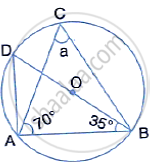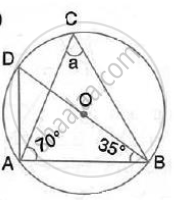Share

In the Following Figures, O is the Centre of the Circle. Find the Value of A, B, C and D. - ICSE Class 10 - Mathematics

ConceptChord Properties - Chords Equidistant from the Center Are Equal (Without Proof)

Question

In the following figures, O is the centre of the circle. Find the value of a, b, c and d.SolutionHere, ∠BAD = 90° (Angle in a semicircle)
∴ ∠BDA =90° -35°=55°
Again, a ∠ACB =∠BDA = 55°
(Angle subtended by the same chord on the circle are equal)

Is there an error in this question or solution?

Video TutorialsVIEW ALL 

Solution In the Following Figures, O is the Centre of the Circle. Find the Value of A, B, C and D. Concept: Chord Properties - Chords Equidistant from the Center Are Equal (Without Proof).
S